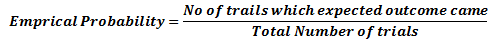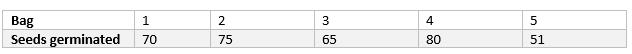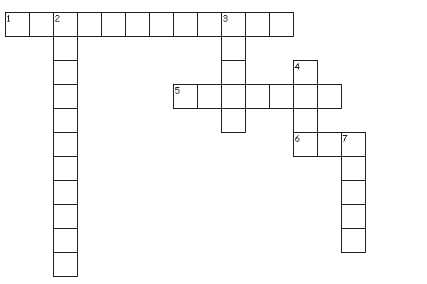# Probability Class 9 Maths notes

Welcome to Class 9 Maths chapter 15 Probability notes.The topics in this page are What is Probability,few terms related to Probability,Empirical Probability and Some Important points about Probability.This is according to CBSE and the NCERT textbook. If you like the study material, feel free to share the link as much as possible.

Table of content

## What is Probability ?

Probability is the measure of the likeliness that an event will occur.Probability is quantified as a number between 0 and 1 (where 0 indicates impossibility and 1 indicates certainty)

## Why we need Probability and what is the use of it?

It is widely used in the study of Mathematics, Statistics, Gambling, Physical sciences, Biological sciences, Weather forecasting, Finance etc. to draw conclusions. Insurance companies uses this to decide on financial policies

## There are few terms related to Probability which are defined below

### Randomness

In mathematics, When next outcome of the experiment can not be determined then we say it is a random experiment e.g. Consider the dice. When we throw the dice, we cannot determine what number will come Since we cannot predict the next outcome, we may say it is a random experiment

### Trial

A trial is an action which results in one or several outcomes, for example each toss of the coin and each throw of the die are called trials

### Independent Trial

Successive trials of some random event for example tosses of a coin,throws of a die are said to be independent if the outcome of any one trial does not impact the outcomes of any others.

### Experiment

Experiment and trial are same thing as such.An experiment is a situation involving chance or probability that leads to results called outcomes.But sometimes we use experiment to refer to whole large number of trials.

### Event

An event is a possible outcome of the Experiment. Like head coming in a toss

### Sample space

It is a set of all possible outcomes of an experiment.

1. when we coin is tossed,the possible outcome are Head and Tail.So sample space
$S={H,T}$
2. When a dice is thrown , the possible outcome is 1,2,3,4,5,6. So Sample space
$S={1,2,3,4,5,6}$

## Empirical Probability

1. Experimental or empirical probability is an estimate that an event will happen based on how often the event occurs after performing an experiment in a large number of trials.
2. It is a probability of event which is calculated based on experiments3. Empirical probability depends on experiment and different will get different values based on the experiment

## Some Important points

1. If the event A, B, C covers the entire possible outcome in the experiment. Then,
$P (A) +P (B) +P(C) =1$
2. The probability of an event (U) which is impossible to occur is 0. Such an event is called an impossible event
P (U)=0
3. The probability of an event (X) which is sure (or certain) to occur is 1. Such an event is called a sure event or a certain event
$P(X) =1$
4. Probability of any event can be as
$0 \geq P(E) \geq 1$

## Solved Examples

Question 1
A coin is tossed 1000 times; we get 499 times head and 501 times tail
a. What is the empirical probability of getting head ?
b. What is the empirical probability of getting tail?
c. Does the sum of above two probability equals 1?
Solution
a. $Empirical \; probability \; of \; getting \; head=\frac {(No \; of \; trails \; which \; heads \; came)}{(Total \; Number \; of \; trials)}$

So empirical or experimental probability of getting head P(H) is calculated as
=.499
b. $Empirical \; probability \; of \; getting \; Tail=\frac {(No \; of \; trails \; which \; tail \; came)}{(Total \; Number \; of \; trials)}$
So empirical or experimental probability of getting head P(T) is calculated as

=.501

c. Now P(H) +P(T)= .499+.501=1
As tail and head are the only possible outcome,the sum of probabilities is 1
Question 2
The record of a weather station shows that out of the past 250 consecutive days, its weather forecasts were correct 175 times.
(i) What is the probability that on a given day it was correct?
(ii) What is the probability that it was not correct on a given day?
Solution
i. Probability that on a given day it was correct = No of day it was correct/Total number of days
=127/250=.7
ii. Number of days it was incorrect=250-175=75
probability that it was not correct on a given day=No of day it was incorrect/Total number of days
=75/250=.3
Question 3
100 seeds were selected at random from each of 5 bags of seeds, and were kept under standardised conditions favourable to germination. After 20 days, the number of seeds which had germinated in each collection were counted and recorded as follows:What is the probability of germination of
(i) more than 60 seeds in a bag?
(ii) 51 seeds in a bag?
(iii) more that 75 seeds in a bag?
(iv) 90 seeds in a bag?
Solution
Total number of bags is 5.
(i) Number of bags in which more than 60 seeds germinated out of 50 seeds is 4.
P(germination of more than 60 seeds in a bag) = 4/5
(ii) P(51 seeds in a bag) =1/5
(iii) P(more that 75 seeds in a bag) =1/5
(iv) P(90 seeds in a bag) =0/5 =0

Crossword PuzzleAcross
1. probability based on outcome of trials
5. Another six letter word of Probability
6. probability of sure event
Down
2. The numerical measure of prediction
3. ______is an action which results in one or several outcomes
4. Probability of impossible event
7. _____ for an experiment is the collection of some outcomes of the experiment.

## Summary

Here is the Probability Class 9 Maths notes Summary
• An event is a possible outcome of the Experiment.
• Probability is the measure of the likeliness that an event will occur
• Emprical Probability is defined as• Probability lies between 0 and 1 with 0 and 1 inclusive

• Notes
• NCERT Solutions & Assignments